Foxtable(狐表)用户栏目专家坐堂 → 不自动递增

共有280人关注过本帖树形打印复制链接

# 主题：不自动递增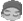nuoyan88
1楼 | 信息 | 搜索 | 邮箱 | 主页 | UC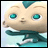加好友发短信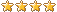代码一：     If Tables("刀具入库申请_单刀明细").current.IsNull("入库日期")  Then        Tables("刀具入库申请_单刀明细").current("单刀编码") = Nothing    Else        Tables("刀具入库申请_单刀明细").current.save        Dim bh As String  = "PC" &  Format(Tables("刀具入库申请_单刀明细").current("入库日期"),"yyMMdd")  ' 取得编号的8位前缀        If Tables("刀具入库申请_单刀明细").current("单刀编码").StartsWith(bh) = False '如果编号的前8位不符            Dim dt2 As DataTable = DataTables("库存明细记录")            Dim max As String            Dim idx As Integer            max = Tables("库存明细记录").DataTable.SQLCompute("Max(单刀编码)","入库日期 = '" &  Tables("刀具入库申请_单刀明细").current("入库日期") & "' And [_Identify] <> " & Tables("刀具入库申请_单刀明细").current("_Identify") & " and 单刀编码 like 'PC%'") '取得该天的最大编号            If max > "" Then '如果存在最大编号                idx = CInt(max.Substring(8,2)) + 1 '获得最大编号的后三位顺序号,并加1            Else                idx = 1 '否则顺序号等于1            End If            Tables("刀具入库申请_单刀明细").current("单刀编码") = bh & Format(idx,"00")            Tables("刀具入库申请_单刀明细").current.save        End If    End If 代码二： Dim t As Table = Forms("刀具入库申请").Controls("单刀明细").TableFor Each r As Row In Tables("刀具入库申请_录入").GetCheckedRows    Dim r2 As Row  = t.AddNew    r2("数量") = r("入库数量")    r2("刀具代码") = r("刀具代码")    r2("规格型号") = r("规格型号")    r2("材料编码") = r("成品编码")    r2("材料描述") = r("成品描述")    r2("入库日期") = Date.Today    r2("单位") = r("单位")    r2("目前状态") = "仓库存放"    r2("采购申请号") = r("采购申请号")    r2("采购订单号") = r("采购订单号")    r2("操作人员") = _UserName    r2("库位") = "刀具库"    r2("出库类型") = "批次配置"Next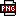此主题相关图片如下：1.png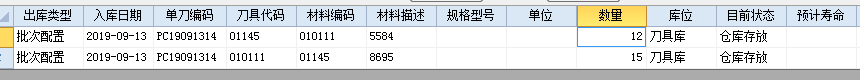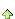2楼 | 信息 | 搜索 | 邮箱 | 主页 | UC加好友发短信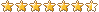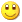Post By：2019/9/15 13:56:00 [只看该作者]

 代码1在什么事件？代码2又在什么事件里？nuoyan88
3楼 | 信息 | 搜索 | 邮箱 | 主页 | UC加好友发短信Post By：2019/9/15 19:05:00 [只看该作者]

 Button类型的控件里4楼 | 信息 | 搜索 | 邮箱 | 主页 | UC加好友发短信Post By：2019/9/15 21:00:00 [只看该作者]

 上传实例测试